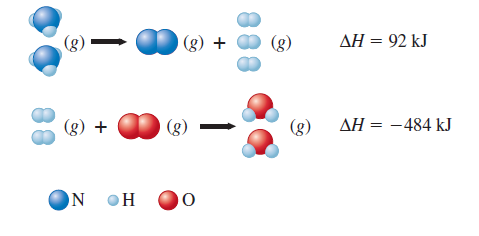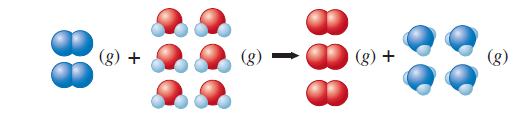# Problem: Given the following data:Calculate ΔH for the reaction On the basis of the enthalpy change, is this a useful reaction for the synthesis of ammonia?

###### FREE Expert Solution

We start solving this by setting up the equations according to the structures drawn

(1) 2NH3 (g) → N2 (g) + 3H(g)    ΔH°rxn = 92 kJ

(2) 2H2 (g) + O2 (g) → 2H2O (l)    ΔH°rxn = -484 kJ

To find the ΔHrxn of the third reaction, we need to use the Hess's Law where we have to rearrange the given equations to get the desired reaction.

83% (187 ratings)###### Problem Details

Given the following data:Calculate ΔH for the reactionOn the basis of the enthalpy change, is this a useful reaction for the synthesis of ammonia?